# CAT 2019 Question Paper | Quants Slot 2

###### CAT Previous Year Paper | CAT Quants Questions | Question 11

Have you ever found yourself stuck on a topic where even after applying the right concepts you struggle to get to the correct answer? Well, the CAT exam is known to put you through these situations and test you on how you comprehend the basics rather than just memorizing a gazillion formulas. To get a hang of how to steer your online CAT preparation in the right direction, it is important to go through CAT previous year paper. They will help you understand the kind, pattern and difficulty level of questions that you can expect from the CAT Question Paper this year. Ace you preparation and glide through the troughs of the exam smoothly by solving these questions. Start with this geometry question taken from CAT 2019 Question paper right away.

Question 11 : Let A and B be two regular polygons having a and b sides, respectively. If b = 2a and each interior angle of B is 3/2 times each interior angle of A, then each interior angle, in degrees, of a regular polygon with a + b sides is [TITA]

## Best CAT Coaching in Chennai

#### CAT Coaching in Chennai - CAT 2022Limited Seats Available - Register Now!

Sum of the interior angles of a n-sided Polygon = (n-2) x 180
Measure of each interior angle =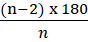So, Interior angle of the polygon with side a =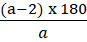Interior angle of the polygon with side b =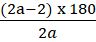It is given that interior angle of side b is 3/2 times to that of the polygon with side A=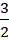x(2a-2) x 180 = (3a - 6) x 180
2a - 2 = 3a - 6
a = 4
So, b = 8
(a + b) sides = 8 + 4 = 12 sides
Interior angle =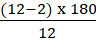= 150o

The question is "Let A and B be two regular polygons having a and b sides, respectively. If b = 2a and each interior angle of B is 3/2 times each interior angle of A, then each interior angle, in degrees, of a regular polygon with a + b sides is [TITA] "

##### Hence, the answer is 150

###### CAT Coaching in ChennaiCAT 2023

Classroom Batches Starting Now! @Gopalapuram

###### Best CAT Coaching in Chennai Introductory offer of 5000/-

Attend a Demo Class

##### Where is 2IIM located?

2IIM Online CAT Coaching
A Fermat Education Initiative,
58/16, Indira Gandhi Street,
Kaveri Rangan Nagar, Saligramam, Chennai 600 093

##### How to reach 2IIM?

Mobile: (91) 99626 48484 / 94459 38484
WhatsApp: WhatsApp Now
Email: info@2iim.com